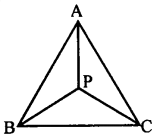# KSEEB Solutions for Class 8 Maths Chapter 11 Congruency of Triangles Ex 11.7

Students can Download Maths Chapter 11 Congruency of Triangles Ex 11.7 Questions and Answers, Notes Pdf, KSEEB Solutions for Class 8 Maths helps you to revise the complete Karnataka State Board Syllabus and score more marks in your examinations.

## Karnataka Board Class 8 Maths Chapter 11 Congruency of Triangles Ex 11.7

Question 1.
In a triangle ABC, ∠B = 28° and ∠C= 56°. Find the largest and smallest side.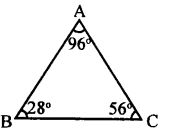∠A + ∠B + ∠C = 180°
[Sum of the angles of a triangle]
∠A + 28 + 56 = 180°
∠A + 84° = 180°
∠A = 180 – 84
∠A = 96°
BC is the largest side
[Opposite to largest angle ∠A]
AC is the smallest side
[Opposite to smalles angle ∠B]

Question 2.
In a triangle ABC, we have AB = 4cm, BC = 5.6 cm and CA = 7.6 cm. Write the angles of the triangle in ascending order
of measures.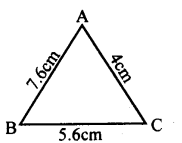∠C <∠A <∠B

Question 3.
Let ΔABC be a triangle such that ∠B = 70° and∠C = 40°. suppose D is a point on BC such that AB = AD. Prove that AB > CD.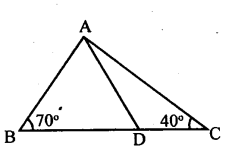In Δ ABD, AB = AD
∴ ∠ABD = ∠ APB = 70° [Theorem]
∠APB +∠ ADC = 180° [Linear pair]
∠DAC = 180 – ( 110 + 40)
= 180 – 150
∠DAC = 30°
∠ACD > ∠DAC
∴ AB > CD

Question 4.
Let ABCD be a quadrilateral in which AD is the largest side and BC is the smallest side. Prove that|A <[C. [Hint: Join AC]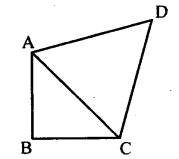Join AC
In Δ ABC   AB > BC [BC is the smallest side]
∴ ∠ACB > ∠BAC …(i)
∠ACD > ∠PAC …(ii)
∠ACB + ∠ACD > ∠BAC + ∠DAC